Editor Ratings:
User Ratings:
[Total: 0 Average: 0]

Google Sheets does not have an in-built word counter. You will have to do some extra work to get the word count using some functions provided by Google Sheets. These functions can help you to count the number of words in a cell, a row, a column, or even the entire sheet.

In this article we will be explaining How you can perform a Word Count in Google Sheets using the native functions.

## Count Words with the SPLIT and COUNTA function

The simplest way to count words automatically in Google Sheets is by using the SPLIT function in conjunction with the COUNTA function. The SPLIT function separates text apart depending on a set delimiter such as ‘space’. SPLIT can also fragment each individual letter if you are also interested in getting a character count alongside a word count. After the SPLIT function breaks the text string into individual words, COUNTA comes into the picture and counts the number of words. The formulas work even if you have double spaces between words or sentences. So, the combination of these two functions gives you a working and very effective word counter.

### How it Works:

1. Navigate to the Sheet where you want to perform a word count and designate a column or cell for the formula.

2. To count words in a Single Cell, copy the formula given below into the designated cell. Replace ‘A3’ with the cell that contains the text.

=COUNTA(SPLIT(A2,” “))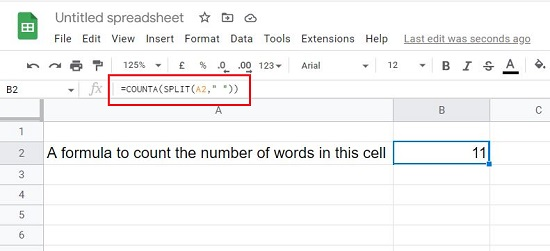3. If you require to perform a word count in multiple cells / rows / columns you must use the ARRAYFORMULA that allows you to calculate a range instead of a single cell. Like earlier, designate a cell where you want to use the formula and place the final word count and copy the formula below into the cell. Replace the range A2:A10 to cover the cell that you need.

=ARRAYFORMULA(SUM(COUNTA(SPLIT(A2:A10,” “))))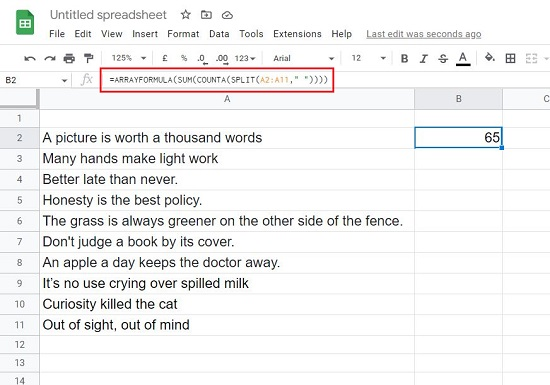4. If you have any blank cell in the specified range, it will be counted as 1 and hence the total word count will be incorrect. To avoid this, you can use the following formula:

=COUNTA(SPLIT(TEXTJOIN(” “, true, A2:A11), ” “))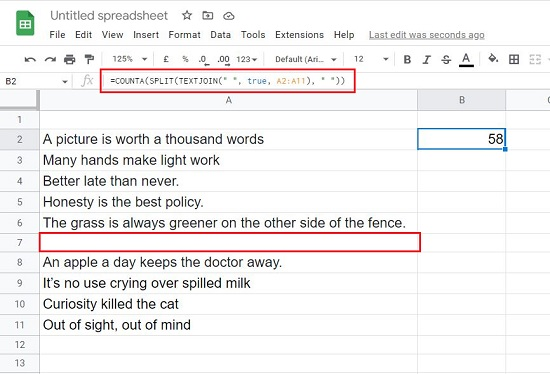5. If you wish to add additional columns to perform a word count, you can simply add a second COUNTA string. For example, if you want to cover column A as well as column C and perform the total word count, you can use the following formula:

=ARRAYFORMULA(COUNTA(SPLIT(A2:A11, ” “))+(COUNTA(SPLIT(C2:C11, ” “))))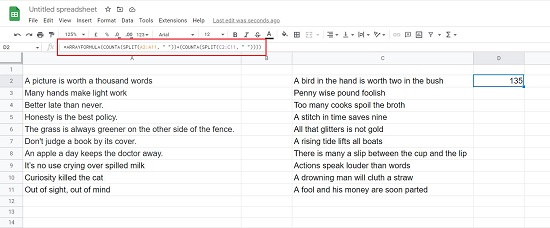6. You can also use the SPLIT function with COUNTIF to only count a specific word or entirely ignore it in your word count. This can be very important if you do not want to repeat a certain word too much in your Google Sheet. You could choose to just count the occurrences of that word and take the necessary action as required. The following formula will count the occurrences of the word ‘you’ in the text string in cell A2. Like earlier, you can modify the cell address to include a range of cells.

=COUNTIF(SPLIT(A2,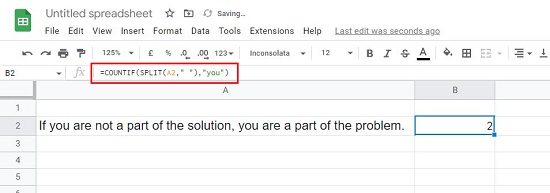” “),”you”))

7. To ignore a certain word, prefix the word with <>. For example, the following formula will count the number of words in cell A2 excepting the word ‘you’.

=COUNTIF(SPLIT(A2,” “),”<>you”))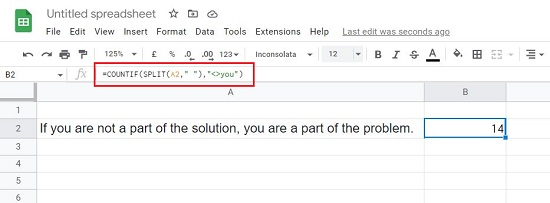## Count Words with the LEN function

SPLIT is the better method to perform a word count in Google Sheets. But the LEN function combined with SUBSTITUTE can also work for you. LEN is primarily used to count the number of characters in a cell or range, but when you combine it with SUBSTITUTE you can simply count the spaces, as for each space there will be a word. Since there is no space used after the last word in a sentence, you will have to add 1 to account for the last word to get an accurate count.

### How it Works:

1. Choose the cell where you want to place your word count and insert the formula given below into the cell.

=LEN(A2)-LEN(SUBSTITUTE(A2,” “,””))+1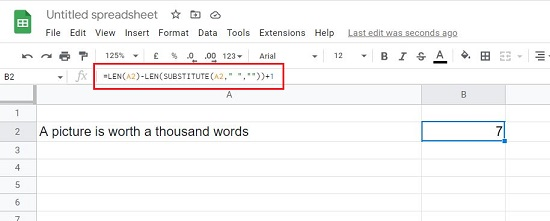2. If you WANT to perform a word count in a range of cells using LEN you must use the SUMPRODUCT formula. Instead of adding at the end of the formula, you must add a number equal to the number of cells in the range. For example, for the range A2:A13, you must add 12 to get the accurate word count. See the example below.

=SUMPRODUCT(LEN(A2:A13)-LEN(SUBSTITUTE(A2:A13,” “,””)))+12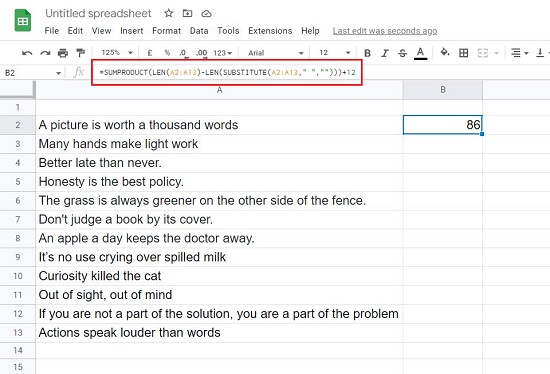The primary problem with the LEN formula is with the presence of accidental double spaces between words. The extra space counts as a word and hence you will get an improper count of words.